# Colormap Normalizations¶

Demonstration of using norm to map colormaps onto data in non-linear ways.

import numpy as np
import matplotlib.pyplot as plt
import matplotlib.colors as colors


Lognorm: Instead of pcolor log10(Z1) you can have colorbars that have the exponential labels using a norm.

N = 100
X, Y = np.mgrid[-3:3:complex(0, N), -2:2:complex(0, N)]

# A low hump with a spike coming out of the top.  Needs to have
# z/colour axis on a log scale so we see both hump and spike.  linear
# scale only shows the spike.

Z1 = np.exp(-X**2 - Y**2)
Z2 = np.exp(-(X * 10)**2 - (Y * 10)**2)
Z = Z1 + 50 * Z2

fig, ax = plt.subplots(2, 1)

pcm = ax.pcolor(X, Y, Z,
norm=colors.LogNorm(vmin=Z.min(), vmax=Z.max()),
cmap='PuBu_r')
fig.colorbar(pcm, ax=ax, extend='max')

pcm = ax.pcolor(X, Y, Z, cmap='PuBu_r')
fig.colorbar(pcm, ax=ax, extend='max')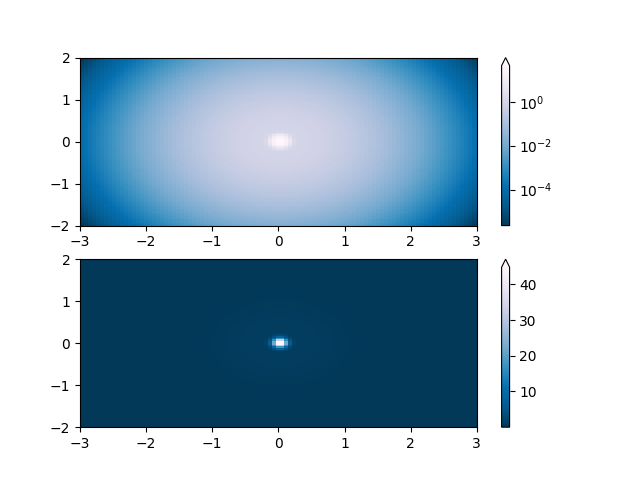Out:

/root/matplotlib/examples/userdemo/colormap_normalizations.py:30: MatplotlibDeprecationWarning: shading='flat' when X and Y have the same dimensions as C is deprecated since 3.3.  Either specify the corners of the quadrilaterals with X and Y, or pass shading='auto', 'nearest' or 'gouraud', or set rcParams['pcolor.shading'].  This will become an error two minor releases later.
pcm = ax.pcolor(X, Y, Z,
/root/matplotlib/examples/userdemo/colormap_normalizations.py:35: MatplotlibDeprecationWarning: shading='flat' when X and Y have the same dimensions as C is deprecated since 3.3.  Either specify the corners of the quadrilaterals with X and Y, or pass shading='auto', 'nearest' or 'gouraud', or set rcParams['pcolor.shading'].  This will become an error two minor releases later.
pcm = ax.pcolor(X, Y, Z, cmap='PuBu_r')

<matplotlib.colorbar.Colorbar object at 0x7f08b48da070>


PowerNorm: Here a power-law trend in X partially obscures a rectified sine wave in Y. We can remove the power law using a PowerNorm.

X, Y = np.mgrid[0:3:complex(0, N), 0:2:complex(0, N)]
Z1 = (1 + np.sin(Y * 10.)) * X**2

fig, ax = plt.subplots(2, 1)

pcm = ax.pcolormesh(X, Y, Z1, norm=colors.PowerNorm(gamma=1. / 2.),
cmap='PuBu_r')
fig.colorbar(pcm, ax=ax, extend='max')

pcm = ax.pcolormesh(X, Y, Z1, cmap='PuBu_r')
fig.colorbar(pcm, ax=ax, extend='max')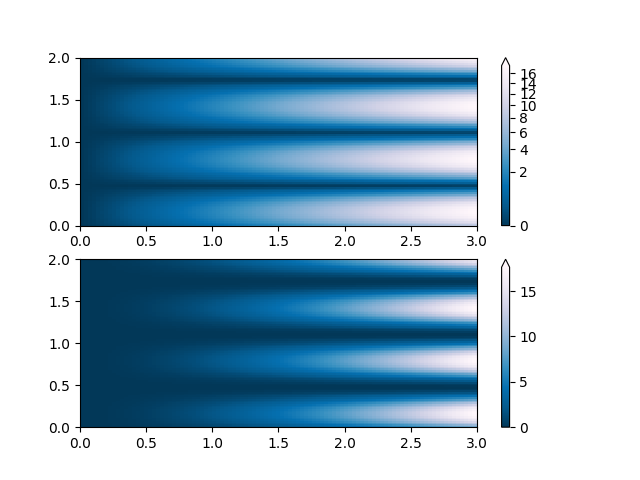Out:

/root/matplotlib/examples/userdemo/colormap_normalizations.py:48: MatplotlibDeprecationWarning: shading='flat' when X and Y have the same dimensions as C is deprecated since 3.3.  Either specify the corners of the quadrilaterals with X and Y, or pass shading='auto', 'nearest' or 'gouraud', or set rcParams['pcolor.shading'].  This will become an error two minor releases later.
pcm = ax.pcolormesh(X, Y, Z1, norm=colors.PowerNorm(gamma=1. / 2.),
/root/matplotlib/examples/userdemo/colormap_normalizations.py:52: MatplotlibDeprecationWarning: shading='flat' when X and Y have the same dimensions as C is deprecated since 3.3.  Either specify the corners of the quadrilaterals with X and Y, or pass shading='auto', 'nearest' or 'gouraud', or set rcParams['pcolor.shading'].  This will become an error two minor releases later.
pcm = ax.pcolormesh(X, Y, Z1, cmap='PuBu_r')

<matplotlib.colorbar.Colorbar object at 0x7f08b47959d0>


SymLogNorm: two humps, one negative and one positive, The positive with 5-times the amplitude. Linearly, you cannot see detail in the negative hump. Here we logarithmically scale the positive and negative data separately.

Note that colorbar labels do not come out looking very good.

X, Y = np.mgrid[-3:3:complex(0, N), -2:2:complex(0, N)]
Z1 = 5 * np.exp(-X**2 - Y**2)
Z2 = np.exp(-(X - 1)**2 - (Y - 1)**2)
Z = (Z1 - Z2) * 2

fig, ax = plt.subplots(2, 1)

pcm = ax.pcolormesh(X, Y, Z1,
norm=colors.SymLogNorm(linthresh=0.03, linscale=0.03,
vmin=-1.0, vmax=1.0, base=10),
cmap='RdBu_r')
fig.colorbar(pcm, ax=ax, extend='both')

pcm = ax.pcolormesh(X, Y, Z1, cmap='RdBu_r', vmin=-np.max(Z1))
fig.colorbar(pcm, ax=ax, extend='both')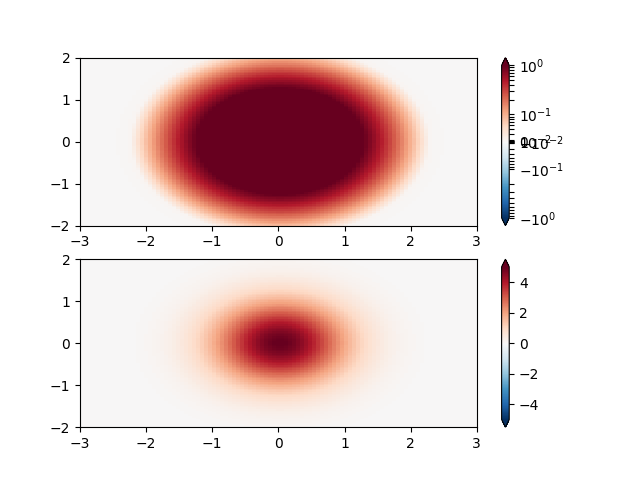Out:

/root/matplotlib/examples/userdemo/colormap_normalizations.py:70: MatplotlibDeprecationWarning: shading='flat' when X and Y have the same dimensions as C is deprecated since 3.3.  Either specify the corners of the quadrilaterals with X and Y, or pass shading='auto', 'nearest' or 'gouraud', or set rcParams['pcolor.shading'].  This will become an error two minor releases later.
pcm = ax.pcolormesh(X, Y, Z1,
/root/matplotlib/examples/userdemo/colormap_normalizations.py:76: MatplotlibDeprecationWarning: shading='flat' when X and Y have the same dimensions as C is deprecated since 3.3.  Either specify the corners of the quadrilaterals with X and Y, or pass shading='auto', 'nearest' or 'gouraud', or set rcParams['pcolor.shading'].  This will become an error two minor releases later.
pcm = ax.pcolormesh(X, Y, Z1, cmap='RdBu_r', vmin=-np.max(Z1))

<matplotlib.colorbar.Colorbar object at 0x7f08b45ae250>


Custom Norm: An example with a customized normalization. This one uses the example above, and normalizes the negative data differently from the positive.

X, Y = np.mgrid[-3:3:complex(0, N), -2:2:complex(0, N)]
Z1 = np.exp(-X**2 - Y**2)
Z2 = np.exp(-(X - 1)**2 - (Y - 1)**2)
Z = (Z1 - Z2) * 2

# Example of making your own norm.  Also see matplotlib.colors.
# From Joe Kington: This one gives two different linear ramps:

class MidpointNormalize(colors.Normalize):
def __init__(self, vmin=None, vmax=None, midpoint=None, clip=False):
self.midpoint = midpoint
colors.Normalize.__init__(self, vmin, vmax, clip)

def __call__(self, value, clip=None):
# I'm ignoring masked values and all kinds of edge cases to make a
# simple example...
x, y = [self.vmin, self.midpoint, self.vmax], [0, 0.5, 1]
return np.ma.masked_array(np.interp(value, x, y))

#####
fig, ax = plt.subplots(2, 1)

pcm = ax.pcolormesh(X, Y, Z,
norm=MidpointNormalize(midpoint=0.),
cmap='RdBu_r')
fig.colorbar(pcm, ax=ax, extend='both')

pcm = ax.pcolormesh(X, Y, Z, cmap='RdBu_r', vmin=-np.max(Z))
fig.colorbar(pcm, ax=ax, extend='both')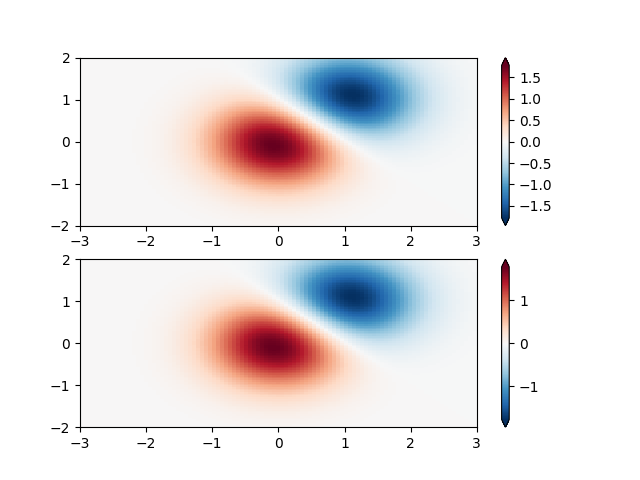Out:

/root/matplotlib/examples/userdemo/colormap_normalizations.py:109: MatplotlibDeprecationWarning: shading='flat' when X and Y have the same dimensions as C is deprecated since 3.3.  Either specify the corners of the quadrilaterals with X and Y, or pass shading='auto', 'nearest' or 'gouraud', or set rcParams['pcolor.shading'].  This will become an error two minor releases later.
pcm = ax.pcolormesh(X, Y, Z,
/root/matplotlib/examples/userdemo/colormap_normalizations.py:114: MatplotlibDeprecationWarning: shading='flat' when X and Y have the same dimensions as C is deprecated since 3.3.  Either specify the corners of the quadrilaterals with X and Y, or pass shading='auto', 'nearest' or 'gouraud', or set rcParams['pcolor.shading'].  This will become an error two minor releases later.
pcm = ax.pcolormesh(X, Y, Z, cmap='RdBu_r', vmin=-np.max(Z))

<matplotlib.colorbar.Colorbar object at 0x7f08b43a3be0>


BoundaryNorm: For this one you provide the boundaries for your colors, and the Norm puts the first color in between the first pair, the second color between the second pair, etc.

fig, ax = plt.subplots(3, 1, figsize=(8, 8))
ax = ax.flatten()
# even bounds gives a contour-like effect
bounds = np.linspace(-1, 1, 10)
norm = colors.BoundaryNorm(boundaries=bounds, ncolors=256)
pcm = ax.pcolormesh(X, Y, Z,
norm=norm,
cmap='RdBu_r')
fig.colorbar(pcm, ax=ax, extend='both', orientation='vertical')

# uneven bounds changes the colormapping:
bounds = np.array([-0.25, -0.125, 0, 0.5, 1])
norm = colors.BoundaryNorm(boundaries=bounds, ncolors=256)
pcm = ax.pcolormesh(X, Y, Z, norm=norm, cmap='RdBu_r')
fig.colorbar(pcm, ax=ax, extend='both', orientation='vertical')

pcm = ax.pcolormesh(X, Y, Z, cmap='RdBu_r', vmin=-np.max(Z1))
fig.colorbar(pcm, ax=ax, extend='both', orientation='vertical')

plt.show()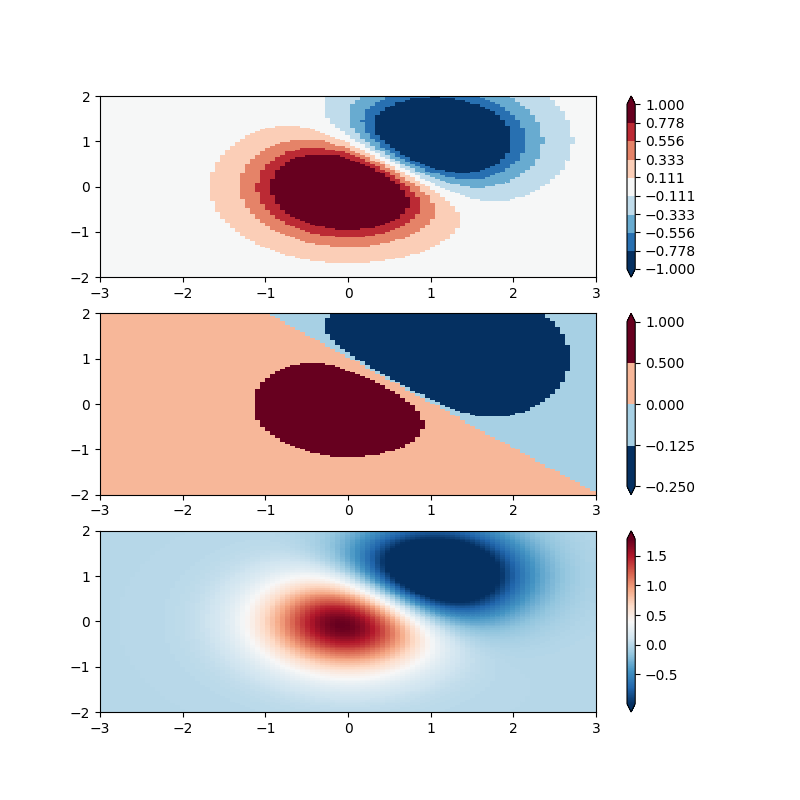Out:

/root/matplotlib/examples/userdemo/colormap_normalizations.py:127: MatplotlibDeprecationWarning: shading='flat' when X and Y have the same dimensions as C is deprecated since 3.3.  Either specify the corners of the quadrilaterals with X and Y, or pass shading='auto', 'nearest' or 'gouraud', or set rcParams['pcolor.shading'].  This will become an error two minor releases later.
pcm = ax.pcolormesh(X, Y, Z,
/root/matplotlib/examples/userdemo/colormap_normalizations.py:135: MatplotlibDeprecationWarning: shading='flat' when X and Y have the same dimensions as C is deprecated since 3.3.  Either specify the corners of the quadrilaterals with X and Y, or pass shading='auto', 'nearest' or 'gouraud', or set rcParams['pcolor.shading'].  This will become an error two minor releases later.
pcm = ax.pcolormesh(X, Y, Z, norm=norm, cmap='RdBu_r')
/root/matplotlib/examples/userdemo/colormap_normalizations.py:138: MatplotlibDeprecationWarning: shading='flat' when X and Y have the same dimensions as C is deprecated since 3.3.  Either specify the corners of the quadrilaterals with X and Y, or pass shading='auto', 'nearest' or 'gouraud', or set rcParams['pcolor.shading'].  This will become an error two minor releases later.
pcm = ax.pcolormesh(X, Y, Z, cmap='RdBu_r', vmin=-np.max(Z1))


Total running time of the script: ( 0 minutes 2.600 seconds)

Keywords: matplotlib code example, codex, python plot, pyplot Gallery generated by Sphinx-Gallery